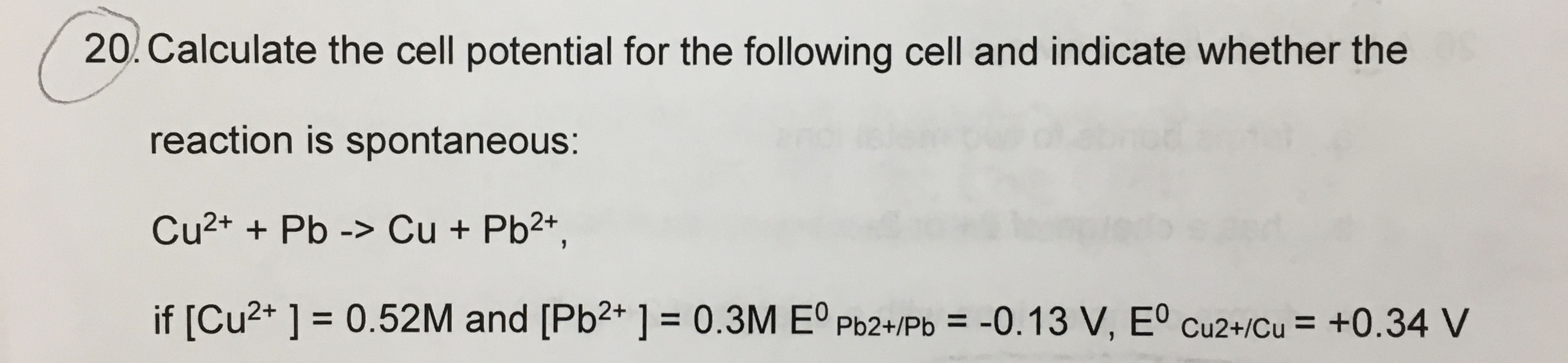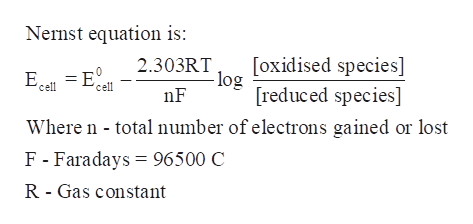# 20. Calculate the cell potential for the following cell and indicate whether thereaction is spontaneous:Cu2+ + Pb -> Cu + Pb2+,if [Cu2+ ] = 0.52M and [Pb2* ] = 0.3M Eº Pb2+/Pb =-0.13 V, Eº cu2+/Cu = +0.34 V%D%3D%3D

Question
8 viewshelp_outlineImage Transcriptionclose20. Calculate the cell potential for the following cell and indicate whether the reaction is spontaneous: Cu2+ + Pb -> Cu + Pb2+, if [Cu2+ ] = 0.52M and [Pb2* ] = 0.3M Eº Pb2+/Pb = -0.13 V, Eº cu2+/Cu = +0.34 V %D %3D %3D fullscreen
check_circle

Step 1

The cell potential for the following cell is to be calculated and indicate whether the reaction is spontaneous or not if

[Cu2+] = 0.52 M, [Pb2+] = 0.3 M

E0 (Pb2+/Pb) = -0.13 V

E0 (Cu2+/Cu) = +0.34 V

Step 2

A reaction is spontaneous if standard electrode potential of the cell, E0Cell, is positive.

Where E0Cell = E0 (cathode) – E0 (anode)

The cathode is where species are reduced and at anode species are oxidised.

Step 3

Nernst equation is used to calculate the ...help_outlineImage TranscriptioncloseNernst equation is: [oxidised species] - log [reduced species] 2.303RT E = E 'cell 'cell nF Where n - total number of electrons gained or lost F - Faradays = 96500 C R - Gas constant fullscreen

### Want to see the full answer?

See Solution

#### Want to see this answer and more?

Solutions are written by subject experts who are available 24/7. Questions are typically answered within 1 hour.*

See Solution
*Response times may vary by subject and question.
Tagged in

### Physical Chemistry